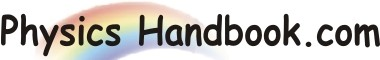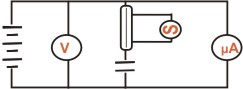HOME TOPICS DEFINITIONS TABLES LAWS INVENTIONS EXPERIMENTS QUIZ VIDEOS
 Capacitance Of A Capacitor Using Reed Switch Aim : The aim of this experiment is to measure the capacitance of a capacitor using a reed switch. Circuit :Materials : -   A Reed Switch -   A Signal Generator -   A Voltmeter -   A Micro-Ammeter -   A Power Supply -   A Set of Capacitors -   Leads Procedure : -   Set up the circuit shown in the diagram. -   Adjust the frequency (f) of the signal generator to give a smooth switching of the reed relay. -   Record the current (I) in the meter. -   Record the voltage (V) across the coil. -   Repeat the experiment for a number of other capacitors. Conclusion : You can calculate the capacitance (C) of the capacitor from, C = I / (f x V)